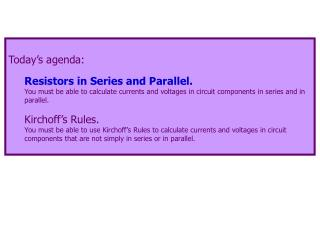# Today’s agenda: Resistors in Series and Parallel. - PowerPoint PPT PresentationDownload PresentationToday’s agenda: Resistors in Series and Parallel.

Today’s agenda: Resistors in Series and Parallel.
Download Presentation## Today’s agenda: Resistors in Series and Parallel.

- - - - - - - - - - - - - - - - - - - - - - - - - - - E N D - - - - - - - - - - - - - - - - - - - - - - - - - - -
##### Presentation Transcript

1. Today’s agenda: Resistors in Series and Parallel. You must be able to calculate currents and voltages in circuit components in series and in parallel. Kirchoff’s Rules. You must be able to use Kirchoff’s Rules to calculate currents and voltages in circuit components that are not simply in series or in parallel.

2. Resistances in Circuits There are “two” ways to connect circuit elements. Series: A B Put your finger on the wire at A. If you can move along the wires to B without ever having a choice of which wire to follow, the circuit components are connected in series. Truth in advertising: it is possible to have circuit elements that are connected neither in series nor in parallel. See problem 24.73 in the 12th edition of our text for an example with capacitors.

3. Parallel: ??? A B Put your finger on the wire at A. If in moving along the wires to B you ever have a choice of which wire to follow, the circuit components are connected in parallel.* *Truth in advertising: actually, the circuit components are not connected in series, and may be connected in parallel.

4. Are these resistors in series or parallel? - + V parallel It matters where you put the source of emf.

5. Are these resistors in series or parallel? - V + series It matters where you put the source of emf.

6. If resistors “see” the same potential difference, they are in parallel. If resistors “see” the same current, they are in series. V - + - I V + V parallel series It’s difficult to come up with a simple one- or two-sentence rule for series/parallel.

7. Here’s a circuit with three resistors and a battery: I I I R1 R2 R3 V1 V2 V3 - + V I Current flows… …in the steady state, the same current flows through all resistors… …there is a potential difference (voltage drop) across each resistor.

8. Applying conservation of energy allows us to calculate the equivalent resistance of the series resistors. I am including the derivation in these notes, for the benefit of students who want to look at it. In lecture, I will skip ahead past the derivation.

9. I I I R1 R2 R3 V1 V2 V3 - + I V An electric charge q is given a potential energy qV by the battery. As it moves through the circuit, the charge loses potential energy qV1 as it passes through R1, etc. The charge ends up where it started, so the total energy lost must equal the initial potential energy input: qV = qV1 + qV2 + qV3 .

10. I I I R1 R2 R3 V1 V2 V3 - + I V qV = qV1 + qV2 + qV3 V = V1 + V2 + V3 V = IR1 + IR2 + IR3 Now imagine replacing the three resistors by a single resistor, having a resistance R such that it draws the same current as the three resistors in series.

11. I Req V - + I V As above: V = IReq From before: V = IR1 + IR2 + IR3 Combining: IReq = IR1 + IR2 + IR3 Req = R1 + R2 + R3 For resistors in series, the total resistance is the sum of the separate resistances.

12. We can generalize this to any number of resistors: (resistors in series) a consequence of conservation of energy R1 R2 R3 - + V Note: for resistors in parallel, Req is always greater than any of the Ri.

13. I1 R1 Here’s another circuit with three resistors and a battery. V I2 R2 Current flows… V …different currents flows through different resistors… R3 I3 V …but the voltage drop across each resistor is the same. - + V I

14. Applying conservation of charge allows us to calculate the equivalent resistance of the parallel resistors. I am including the derivation in these notes, for the benefit of students who want to look at it. In lecture, I will skip ahead past the derivation.

15. I1 R1 In the steady state, the current I “splits” into I1, I2, and I3 at point A. V I2 R2 A B I1, I2, and I3 “recombine” to make a current I at point B. V R3 I3 Therefore, the net current flowing out of A and into B is I = I1 + I2 + I3 . V - + V I I Because the voltage drop across each resistor is V:

16. I Req Now imagine replacing the three resistors by a single resistor, having a resistance R such that it draws the same current as the three resistors in parallel. A B V - + V I I From above, I = I1 + I2 + I3, and So that

17. Dividing both sides by V gives We can generalize this to any number of resistors: (resistors in parallel) a consequence of conservation of charge Note: for resistors in parallel, Req is always less than any of the Ri.

18. Summary: Series B A same I, V’s add “just like” capacitors NOT “just like” capacitors Parallel A B same V, I’s add “just like” capacitors NOT “just like” capacitors